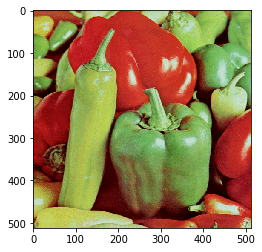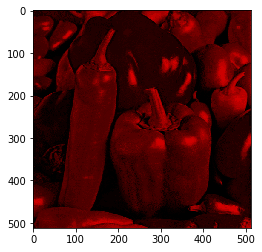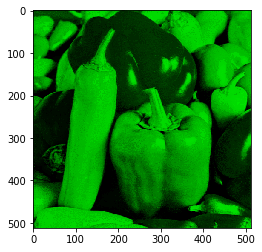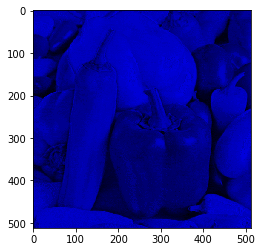# Using Python Splitting Colored Image in to Red, Blue & Green Component## Re integrating the Red, Blue and Green Component into Original Image¶

Image is nothing but a numeric matrix stored in the memory. Each colored Image is a combination of three componenets i.e. Red, Green & Blue. Suppose we have an image of lets say 200 X 300 resolution. The corresponding to that image three matrixes of size 200 X 300 will be stored in the memory each representing Red, Green & Blue component of the image. The values in the matrixes are in the range 0 to 255 wherein 0 represents least intensity of color and 255 represents maximum intensity of the color.

Below are the steps for writing the code to read the colored image and display it using matplotlib.

Step 1 : Import the required libraries. Here we will be using three python libraries cv2 (OpenCV), numpy and matplotlib

Step 2 : Read the image in to 3 dimesnional matrix using opencv imread function

Step 3 : Since, OpenCV takes BGR format and matplotlib takes RGB format. So, if we want to view the image read by OpenCV then we need to convert BGR format in to RGB Format

Step 4: View the RGB Format Image by using imshow() function of matplotlib

In :
```import cv2
import numpy as np
from matplotlib import pyplot as plt
b,g,r = cv2.split(img)       # get b,g,r
rgb_img = cv2.merge([r,g,b])
plt.imshow(rgb_img)
```
Out:
`<matplotlib.image.AxesImage at 0x27844644160>`Now we will break down the three dimensional matrix rgb_img in to three different components Red, Green and Blue.Below are the steps to do the same:

Step 1 : Get the size of three dimsnional matrix rgb_img

Step 2 : Declare three new matrixes corresponding to each color with size as of original image or matrix

Step 3 : Iterate for ‘x’ and ‘y’ axis (2 Dimesnions) to populate values in respective matrixes

Step 4 : Populate first Value in Red

Step 5 : Populate second value in Green

Step 6 : Populae third value in Blue

Step 7 : Display Each Matrix or Image using matplotlib imshow() function

In :
```x,y,z = np.shape(img)
red = np.zeros((x,y,z),dtype=int)
green = np.zeros((x,y,z),dtype=int)
blue = np.zeros((x,y,z),dtype=int)
for i in range(0,x):
for j in range(0,y):
red[i][j] = rgb_img[i][j]
green[i][j]= rgb_img[i][j]
blue[i][j] = rgb_img[i][j]
```
In :
```plt.imshow(red)
```
Out:
`<matplotlib.image.AxesImage at 0x2784469a780>`In :
```plt.imshow(green)
```
Out:
`<matplotlib.image.AxesImage at 0x278446f6390>`In :
```plt.imshow(blue)
```
Out:
`<matplotlib.image.AxesImage at 0x27844751080>`Now we Will try to re create the orignal image from the Red, Green A=and Blue Component of the image.

Steps for the same are as follows:

Step 1 : Declare a three dimensional matrix of type integer of the size of original image

Step 2 : Again iterate through ‘x’and ‘y’ axis (2 Dimesnions)

Step 3 : In the first index populate the value in Red Matrix

Step 4 : In the second index populate the value in Green Matrix

Step 5 : In the third index populate the value in Blue Matrix

Step 6 : Use the imwrite() function of OpenCV to save the image back to disk

Step 7 : Use the imshow() funciton of matplotlib to view the re created image

In :
```#Now we will again create the original image from these Red, Blue and Green Images
retrack_original = np.zeros((x,y,z),dtype=int)
for i in range(0,x):
for j in range(0,y):
retrack_original[i][j] = red[i][j]
retrack_original[i][j] = green[i][j]
retrack_original[i][j] = blue[i][j]
cv2.imwrite('ori.jpg',retrack_original)
plt.imshow(retrack_original)
```
Out:
`<matplotlib.image.AxesImage at 0x27844804d68>`In [ ]:
```
```
In [ ]:
```
```
In [&nbsp;]:
```
```